### Home > A2C > Chapter 8 > Lesson 8.2.2 > Problem8-136

8-136.
1. Find the value of x in each triangle. Homework Help ✎

1.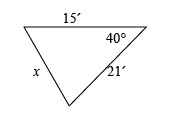2.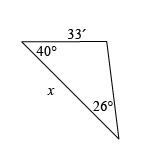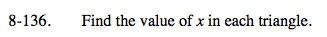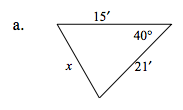Use the Law of Cosines.

C2 = A2 + B2 − 2ABcos(c)
(x)2 = (15)2 + (21)2 − 2(15)(21)cos(40°)

$x=\sqrt{666-630\text{cos}(40\degree)}$

x ≈ 13.542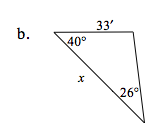Find the last angle, then use the Law of Sines.

x ≈ 68.770